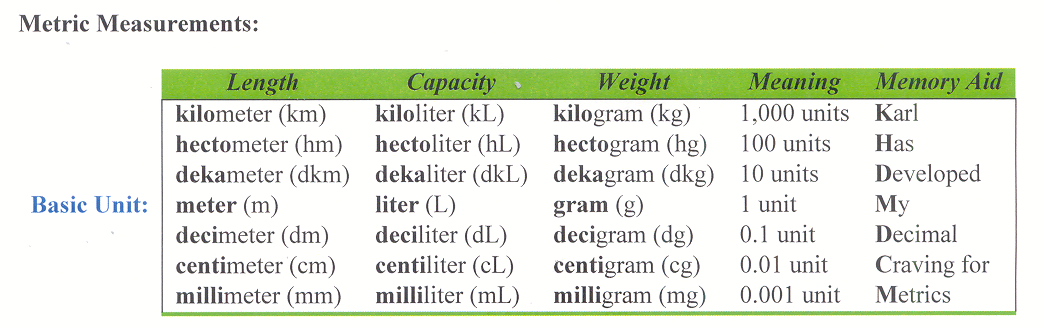# Section 8.4: Miscellaneous Measurements

## 8.4 Outline

1. Review
1. length
2. area
3. volume
2. Volume of solids
1. right rectangular prism
2. right circular cylinder
3. pyramid
4. right circular cone
5. sphere
3. Comparisons
4. Mass
1. definition
2. standard weight units
5. Temperature
1. definition
2. standard temperature units
6. Converting units
1. metric
2. U.S.

## 8.4 Essential Ideas

Use linear units to measure length, square units to measure area, and cubic units to measure volume.

To measure mass, the amount of matter an object comprises, use the following units of measurement:

 U.S. System Metric System ounce (oz) gram (g) pound (lb; 16 oz) kilogram (kg; 1,000 g) ton (2,000 lb) milligram (mg; 1/1,000 g)

To measure temperature, use the following units of measurement:

 U.S. System Metric System Fahrenheit (F) Celsius (C)